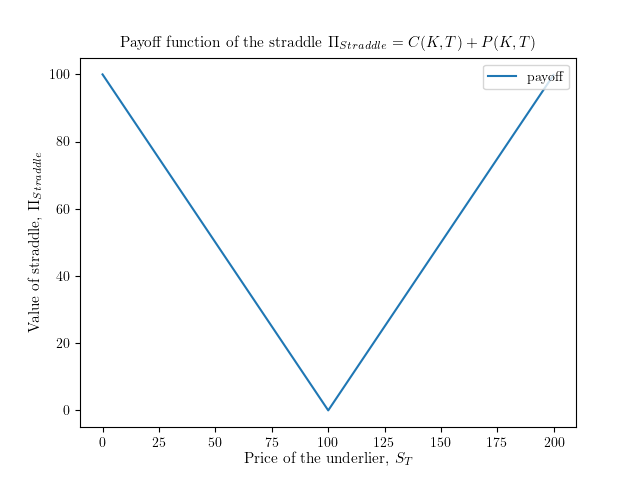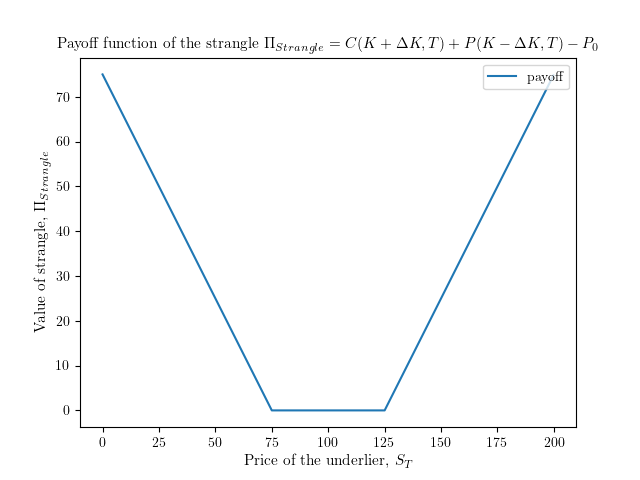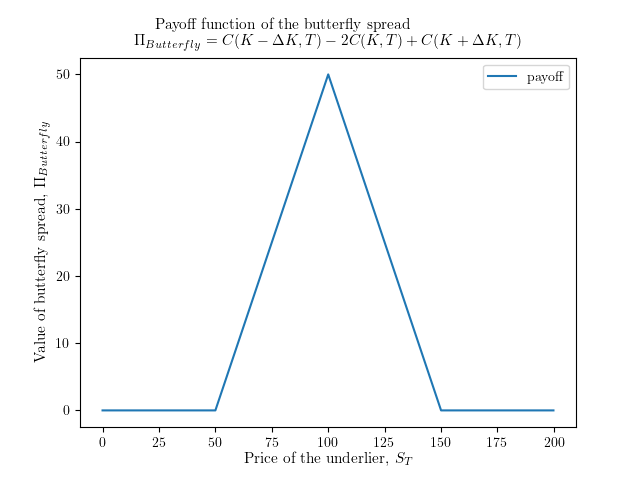📓

# More option spreads

In this article, we cover more complex option strategies that involve options on the same underlying asset at the same expiration, but at different strike prices. In particular, we will discuss call butterfly spreads, straddles, and strangles.

Below we will use the following notation: C(K, T) refers to a call position at strike price and expiration time T, and similarly, P(K, T) refers to a put position with strike price and expiration time T.The long straddle has the payoff function shown above, and is constructed with the call position C(K, T) and put position P(K, T). In this example, K=100, so this strategy would be constructed by going long C(100, T) and long P(100, T).

This strategy is typically used when investors expect the price of the underlier to experience a large change in either direction in a specific period of time, or that the volatility of the underlier will remain high or increase.

## StrangleClosely related to the straddle is the strangle. A long strangle has the payoff function shown above, and is constructed with the call position C(K + ΔK, T) and put position P(K -ΔK, T). In this example, K=100 and ΔK=25, So, this strategy could be constructed by buying both C(125,T) and P(75,T). Note that the premium is at least P₀ = 50.

This strategy is typically used when investors expect the price of the underlier to experience a large change in either direction in a specific period of time, or that the volatility of the underlier will remain high or increase. Compared to the straddle, the strangle costs less but incurs greater risk as the underlying must move a greater amount for the investor to profit.

## Long call butterfly spreadThe butterfly spread has the payoff function shown above and is constructed with the two long call positions C(K -ΔK, T) and C(K + ΔK, T), with ΔK>0 in combination with the short position -2C(K, T). In the example above, K=100 and ΔK=50, so this spread would be constructed by going long (buying) C(50 , T) and C(150, T) and going short (selling) 2 * C(100, T).

This strategy is typically used when investors expect the price of the underlier to remain near its current price within the specified time frame, or that the volatility (the extent to which the price of the underlier is varying with time) will remain low or decrease.